# What is the integer number eight?

## Whole numbers

Here you can learn what whole numbers are (and what not) and how to calculate with them. Let's look at this:

• A Explanationwhat are integers.
• Lots Examples dealing with whole numbers.
• Tasks / exercises so that you can practice this type of number yourself.
• A Video to this topic.
• A Question and answer area to whole numbers.

We'll look at what whole numbers are in a moment. Part of the whole number are the natural numbers. If you want to learn something about these, you can also take a look at the natural numbers.

### Explanation of whole numbers

What are whole numbers? You might think the opposite of "broken" numbers. In principle it is. Because the whole numbers are all the numbers that are "whole" and have no parts behind a decimal point. These are first of all the natural numbers including the zero (0, 1, 2, 3, 4, 5, 6 etc.) and exactly these numbers with a minus sign in front (-1, -2, -3, -4, -5 , -6 etc.)

Note:

Definition and properties of whole numbers:

• The whole numbers include all natural numbers, including zero. These are: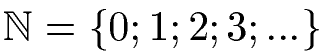• These numbers with a minus sign in front of them also belong to the whole numbers

The whole numbers are summarized with the letter Z, with a double line inserted.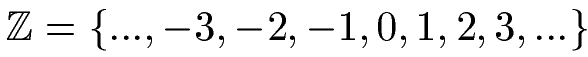example 1:

The next picture shows a series of numbers. Circle each number that is an integer in red. All others should be circled in blue.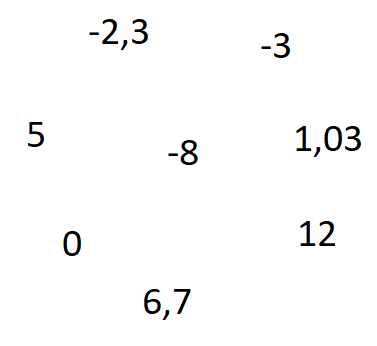Solution:

All numbers that have decimal places are no whole numbers.

All the numbers without Places after the comma, however, are whole numbers.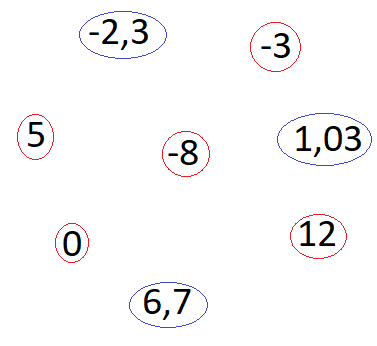Display:

### Examples of whole numbers

In this section we look at a number of examples of integers and how they are used. Let's start with the basic arithmetic operations, i.e. how to add, subtract, multiply and divide whole numbers. Further topics are addressed in the question and answer area.

Example 2:

The addition and subtraction of whole numbers should be shown on the next straight line. These tasks are to be calculated: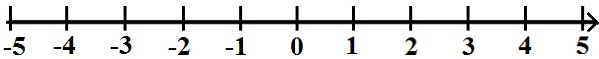Solution:

Let's start with - 5 + 3. This means that we go from -5 by 3 to the right. This brings us to the -2.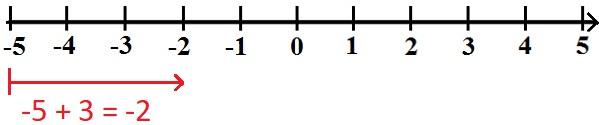Next, let's look at 3-6. This means that we go 6 to the left from the +3. We land on the -3.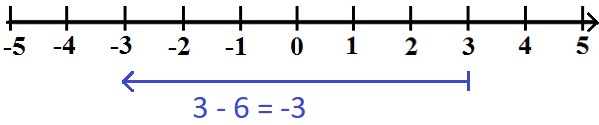We're still missing -1 -4. This means that from the -1 we go 4 to the left. So we land on -5.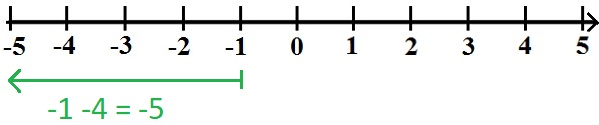Example 3:

Use a calculation to show how negative and positive numbers - namely whole numbers - behave with the signs during multiplication.

Solution:

If one of the two numbers is negative, then the result is also negative. In the other cases the result is positive.

• 5 · 5 = 25
• (-5) · 5 = -25
• 5 · (-5) = -25
• (-5) · (-5) = 25

Example 4:

Use a calculation to show how negative and positive numbers - namely whole numbers - behave with the signs during division.

Solution:

If one of the two numbers is negative, then the result is also negative. In the other cases the result is positive.

• 5 : 5 = 1
• (-5) : 5 = -1
• 5 : (-5) = -1
• (-5) : (-5) = 1

Show:

### Number types explanation

What are the different types of numbers? What are whole numbers and what other types of numbers are important? We'll look at this in the next video. These are the contents:

• Natural numbers
• Negative numbers
• Rational numbers
• Irrational numbers
• Real numbers
• Complex numbers

Next video »

### Questions with answers whole numbers

This section deals with typical questions with answers to whole numbers.

Q: Can you round to whole numbers?

A: You can round to whole numbers. For example, you can round +2.3 to 2 or -5.9 to -6. You can read how this works under rounding numbers (with rules).

Q: is there a largest or smallest integer? How many whole numbers are there?

A: There is no largest or smallest integer, because you can count on as you like: 1, 2, 3, 4, 5, 6, etc. or in the other direction -1, -2, -3, -4, - 5, -6, etc. Hence there are infinitely many whole numbers.

Q: When is this topic covered in school?

A: The subject of whole numbers is mostly dealt with in school from year 5 onwards. The natural numbers (as part of the whole numbers) are already on the agenda in 1st grade. However, since some of the negative numbers also belong to the whole numbers, these follow, as I said, much later.

Q: What other types of numbers are there?

A: Other types of numbers are:

• Natural numbers
• Negative numbers
• Rational numbers
• Irrational numbers.
• Real numbers
• Complex numbers